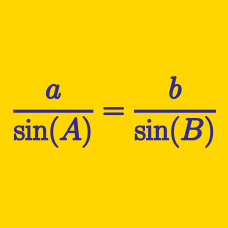Geometry

# Triangle Solving Warmup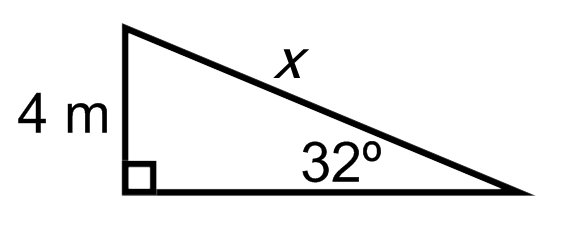What is $$x?$$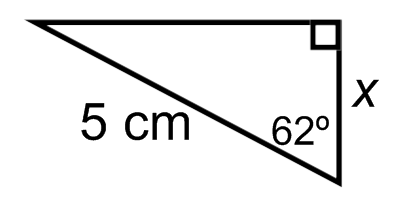What is $$x$$?Which of these is equivalent to $$\cos(a)$$?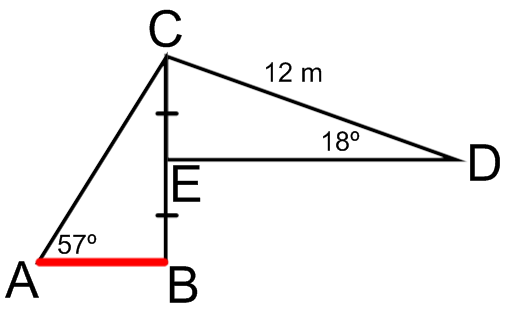What is the length of $$\overline{AB}?$$ (marked in red)

Earlier, we used angles to help solve for side lengths of right triangles. Let's see if we can reason with side lengths to solve for angles.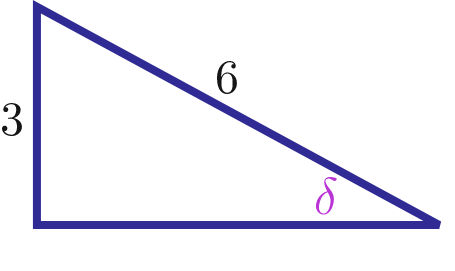What is $$\delta$$ in radians?

×